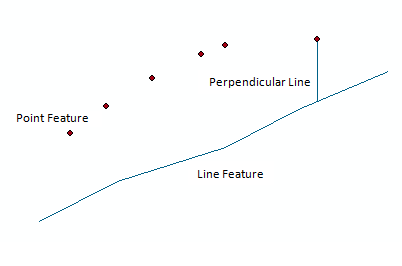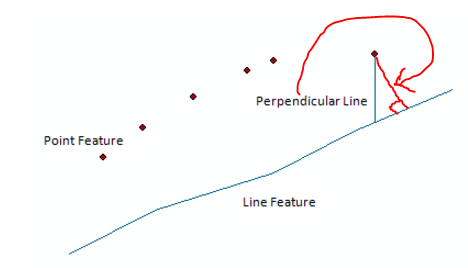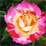# Draw Perpendicular Line.

4836
7
02-25-2016 12:29 AMby
New Contributor

Hello,

Can anyone suggest me to draw the perpendicular line for very point automatically using python/arcobject. That Perpendicular line touch with line feature. Kindly reference the below image.Regards,

Raj

1 Solution

Accepted SolutionsbyEsri Esteemed Contributor

The code below seems to do the job, BUT this is based on a single line. If you have several polylines you will have to include logic to select the polyline to "snap to".```import arcpy

def main():
fc_lines = r'D:\Xander\GeoNet\Perpendicular\data.gdb\lines'
fc_points = r'D:\Xander\GeoNet\Perpendicular\data.gdb\points'
fc_out = r'D:\Xander\GeoNet\Perpendicular\data.gdb\result'
tolerance = 15000

# get first line
polyline = arcpy.da.SearchCursor(fc_lines, ('SHAPE@')).next()

lines = []
with arcpy.da.SearchCursor(fc_points, ('SHAPE@')) as curs:
for row in curs:
pnt = row
line = createVerticalLine(pnt.firstPoint, tolerance, pnt.spatialReference)
mp = getIntersectingPoints(line, polyline)
if mp.pointCount > 0:
pnt2 = getNearestPoint(mp, pnt.firstPoint)
vert_line = arcpy.Polyline(arcpy.Array([pnt.firstPoint, pnt2]),
pnt.spatialReference)
lines.append(vert_line)
else:
# no intersecting within tolerance
pass

arcpy.CopyFeatures_management(lines, fc_out)

def createVerticalLine(pnt, tolerance, sr):
return arcpy.Polyline(arcpy.Array([arcpy.Point(pnt.X, pnt.Y+tolerance),
arcpy.Point(pnt.X, pnt.Y-tolerance)]),
sr)

def getIntersectingPoints(line, polyline):
return polyline.intersect(line, 1)

def getNearestPoint(mp, pnt):
nearest_pnt = None
for p in mp:
if nearest_pnt is None:
nearest_pnt = p
min_dist = getDistance(p, pnt)
else:
dist = getDistance(p, pnt)
if dist < min_dist:
nearest_pnt = p
min_dist = dist
return nearest_pnt

def getDistance(p1, p2):
import math
return math.sqrt((p1.X-p2.X)**2 + (p1.Y-p2.Y)**2)

if __name__ == '__main__':
main()```
7 RepliesbyMVP Esteemed Contributor

Darren Wiens​ posted some code here ​which should get you started.  Your normals are to the X-axis rather than relative to the line.  You can construct an intersecting line relative to that axis which should give you the necessary point intersection on your line and hence the point-line segmentbyEsri Esteemed Contributor

The code below seems to do the job, BUT this is based on a single line. If you have several polylines you will have to include logic to select the polyline to "snap to".```import arcpy

def main():
fc_lines = r'D:\Xander\GeoNet\Perpendicular\data.gdb\lines'
fc_points = r'D:\Xander\GeoNet\Perpendicular\data.gdb\points'
fc_out = r'D:\Xander\GeoNet\Perpendicular\data.gdb\result'
tolerance = 15000

# get first line
polyline = arcpy.da.SearchCursor(fc_lines, ('SHAPE@')).next()

lines = []
with arcpy.da.SearchCursor(fc_points, ('SHAPE@')) as curs:
for row in curs:
pnt = row
line = createVerticalLine(pnt.firstPoint, tolerance, pnt.spatialReference)
mp = getIntersectingPoints(line, polyline)
if mp.pointCount > 0:
pnt2 = getNearestPoint(mp, pnt.firstPoint)
vert_line = arcpy.Polyline(arcpy.Array([pnt.firstPoint, pnt2]),
pnt.spatialReference)
lines.append(vert_line)
else:
# no intersecting within tolerance
pass

arcpy.CopyFeatures_management(lines, fc_out)

def createVerticalLine(pnt, tolerance, sr):
return arcpy.Polyline(arcpy.Array([arcpy.Point(pnt.X, pnt.Y+tolerance),
arcpy.Point(pnt.X, pnt.Y-tolerance)]),
sr)

def getIntersectingPoints(line, polyline):
return polyline.intersect(line, 1)

def getNearestPoint(mp, pnt):
nearest_pnt = None
for p in mp:
if nearest_pnt is None:
nearest_pnt = p
min_dist = getDistance(p, pnt)
else:
dist = getDistance(p, pnt)
if dist < min_dist:
nearest_pnt = p
min_dist = dist
return nearest_pnt

def getDistance(p1, p2):
import math
return math.sqrt((p1.X-p2.X)**2 + (p1.Y-p2.Y)**2)

if __name__ == '__main__':
main()```byMVP Honored Contributor

Are you looking for a perpendicular line (90 degrees to the line, as I've added below) or a vertical line (as you've drawn)?byMVP Esteemed Contributor

he wants normal to the x-axis rather than your example of normal to the line,by
New Contributor

Hi,

I need vertical line for each point and touch with line feature.

Regards,

Raj POccasional Contributor

IProximityOperator proximity = <<PolylineFeature>>.ShapeCopy as IProximityOperator;

IPoint out_point = proximity.ReturnNearestPoint(<<input point>>, esriSegmentExtension.esriExtendTangentAtFrom);

Try this code, it will create perpendicular lines to given point.byEsri Esteemed Contributor

True, but if you look at the examples you will see that it was not a perpendicular line the OP was looking for.